# Kissing the frog: A mathematician's guide to mating

September 2008

### The 37% rule

The first question we need to answer is, why is the best strategy to throw back some number of frogs, and then kiss the next one that is better than all those you've seen so far? Well, what else could you do? All you know about the frogs that you've seen is how good they are relative to each other, which you can see from the numbers on their backs. When the first frog hops out, you have absolutely no information (the number on its back tells you nothing). You could kiss it, but there's only a 1 in 100 chance that it's really the handsome prince. So you'd be better off waiting until you've seen a few more frogs ... and that's the strategy!

Let's make things a little more general. If there are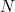frogs in the pond, you will kick back the first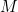frogs, and then kiss the next frog that has a higher number than all those you have seen so far. We have to work out what the best choice ofis. To do that, we have to work out what your chances are of kissing the right frog, depending on where he appears in the sequence:

If the handsome prince is one of the firstfrogs, disaster, you'll kick him back in the pond.

If he is the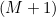th frog, success, you'll kiss him.

If he is the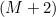th frog, you'll kiss him, unless theth frog had the largest number of all the previous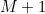frogs. This unfortunate arrangement of frogs, which means that you'll kiss theth frog, not the handsome prince, only occurs in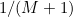of the possible ways of arranging the firstfrogs. (There are just as many arrangements that have the largest numbered frog in the last position, as there are arrangements that have the largest numbered frog in any other position.) You'll kiss the right frog in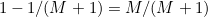of the possible cases.

If he is the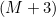th frog, you'll kiss him, unless theth orth frog had the largest number of the previous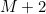frogs. This only occurs in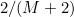of the possible ways of arranging the firstfrogs. You'll kiss the right frog in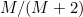of the possible cases.

...

And so on, until

...

If he is theth frog, you'll kiss him, unless theth,th,th,…or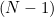th frog had the largest number of the previous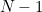frogs. This only occurs in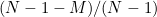of the possible ways of arranging the firstfrogs. You'll kiss the right frog in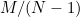of the possible cases.

Now, each of these possible positions of the handsome prince occurs in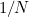of the possible arrangements of the frogs, so your probability of winning is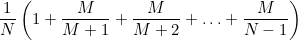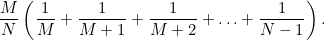For a given total number of frogs, we want to maximise your probability of kissing the handsome prince,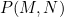, so we want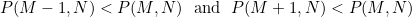starting with the first inequality means that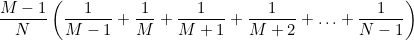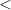Cancelling the factor ofgives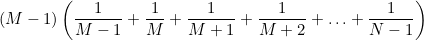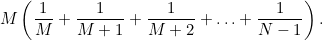Now multiply out the first term of the top line to give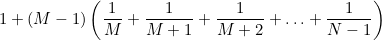Take the right hand side over to the left to give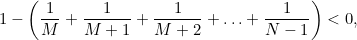and therefore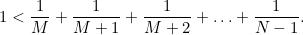We can go through the same calculation for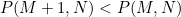(try it!), and find that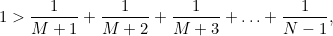so that, finally, we need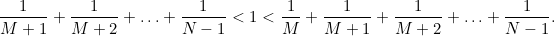We can now work out whatyou should use for various numbers of frogs in the pond (values of). If there are only four frogs, we can see that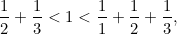so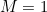, and you should kick back the first frog, and kiss the next one that is better. If there are only five frogs, we can see that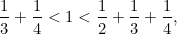so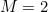, and you should kick back the first two frogs, and kiss the next one that is better. We could continue doing this by hand, but it gets very tedious. Instead, let's define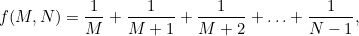and ask a computer to plot this function for us. For 100 frogs (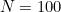), we can see below that you should choose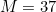.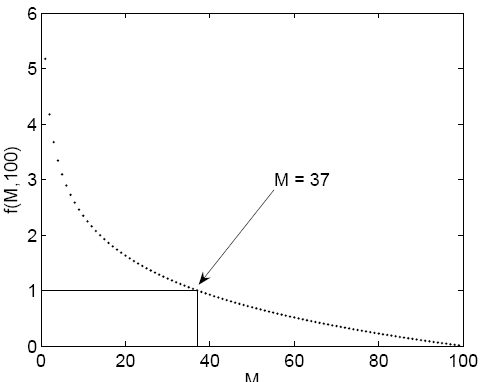For 1000 frogs (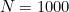), you should choose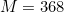.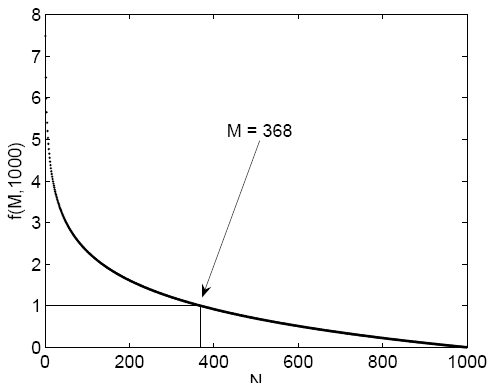Let's plot the value of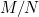that you should choose as a function of.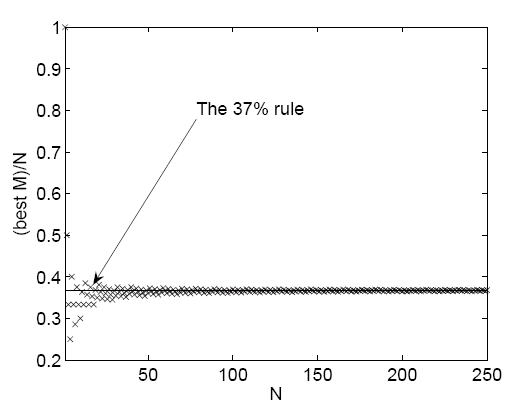Asgets bigger, we can see that the proportion of frogs that you should kick back before thinking about kissing asymptotes to about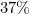. But why? Can we be more precise? Can we estimate the size of the function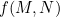whenis large?

Have a look at the graph drawn below.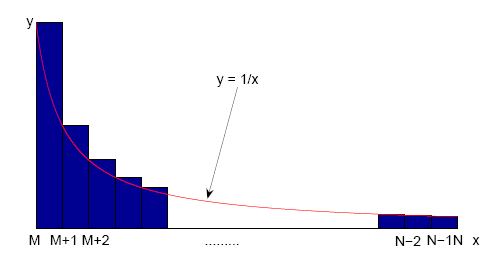The area of the shaded blocks is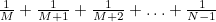. The curve drawn through the top left hand corners of the blocks is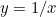. We can see that the area under the curve is less than the area of the blocks, so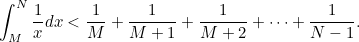But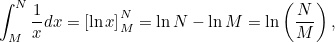so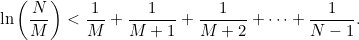Now look at the next graph.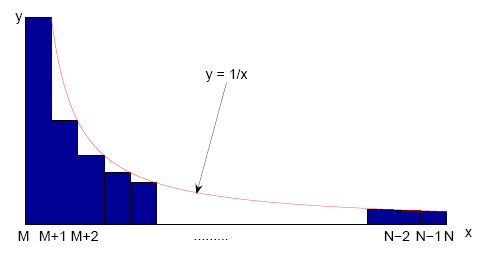In this case, we can see that the area under the blocks (excluding the first block) is less than that under the curve, so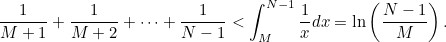Putting this together, we can see that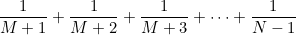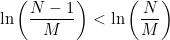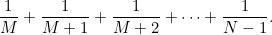However, we are trying to findso that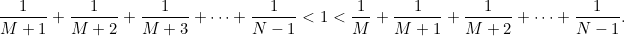Whenandare large,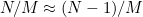, so we need to choosewith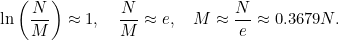But how often does this net you the handsome prince? We found a while ago that the probability of kissing the right frog is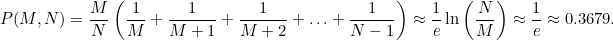So the 37% rule wins the prince 37% of the time, whether there are a hundred, a thousand or a million frogs in the pond. Mind you, it might take a while to kiss 37% of a million frogs. There's another thing to think about in our model!